• 应用回溯法，当可以放置皇后时就继续到下一行，不行的话就返回到第一行，重新检验要放的列数，如此反复，直到将所有解解出。 也就是对于N×N的棋盘，选择出N个符合i!=r∧j!=s∧|i-r|!=|j-s|∨(i+r)!=(j+s)的点的...
• 回溯算法n皇后问题 N-皇后问题 (N - Queen's problem) The n – queen problem is the generalized problem of 8-queens or 4 – queen’s problem. Here, the n – queens are placed on a n * n chess board, ...

回溯算法n皇后问题
N-皇后问题 (N - Queen's problem)
The n – queen problem is the generalized problem of 8-queens or 4 – queen’s problem. Here, the n – queens are placed on a n * n chess board, which means that the chessboard has n rows and n columns and the n queens are placed on thus n * n chessboard such that no two queens are placed in the same row or in the same column or in same diagonal. So that, no two queens attack each other.
n皇后问题是8皇后或4 皇后问题的广义问题 。 在这里，将n –皇后放置在* n棋盘上，这意味着该棋board具有n行和n列，并且将n个皇后放置在n * n棋盘上，这样就不会在同一行中放置两个皇后。在同一列或同一对角线中。 因此，没有两个女王互相攻击。
Here, we suppose that the queen i is to be placed in row i. We can say that 1 queen is placed in the first row only but can have columns from 1, 2... n so that they satisfy all the explicit and implicit constraints.
在这里，我们假设将女王i放在第i行。 我们可以说1个皇后只放在第一行，但是可以有1、2 ... n个列，这样它们就可以满足所有显式和隐式约束。
All solutions to the n – queen’s problem can be therefore represented as n – tuples (x1, x2... xn) where xi is the column on which queen i is to be placed.
因此，n- 皇后问题的所有解决方案都可以表示为n-元组(x1，x2 ... xn)，其中xi是放置女王i的列。
The explicit constraints using this formulation are Si = {1, 2, 3... n-1, n}, where 1 <= I <= n. Therefore, the solution space consists of nˆn n- tuples. Now, while considering the implicit constraints, that no two xi’s can be same i.e., two queens cannot be in the same row, same column, or in same diagonal. So, each xi should be different. Now by the above constraint, the solution is the permutations of the n – tuples (1, 2, 3, 4... n-1, n).
使用此公式的显式约束为Si = {1,2,3 ... n-1，n}，其中1 <= I <= n。 因此，解空间由nˆn个元组组成。 现在，在考虑隐式约束时，没有两个xi可以相同，即两个皇后不能在同一行，同一列或同一对角线中。 因此，每个xi应该不同。 现在，根据上述约束，解决方案是n个元组的排列(1、2、3、4 ... n-1，n)。
算法 (Algorithm )
For all the solutions of the n - queen’s problem...
对于n-皇后问题的所有解...
1.	Algorithm N Queen (k, n)
2.	// Using backtracking, this procedure prints all possible placements of
3.	// n- queens on the n*n chess board so that they are non-attacking.
4.	{
5.	For I = 1 to n do
6.	{
7.	If Place (k, i) then
8.	{
9.	X[k] = I;
10.	If (k = n) then write (x[1: n ]) ;
11.	 Else N Queens (k+1, n);
12.	}
13.	}
14.	}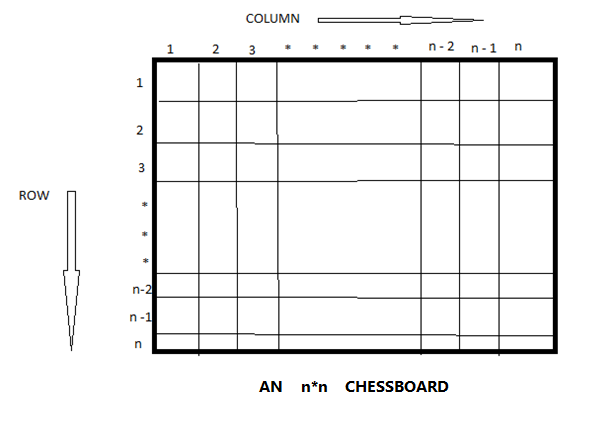翻译自: https://www.includehelp.com/algorithms/n-queens-problem-and-solution-using-backtracking-algorithm.aspx

回溯算法n皇后问题

展开全文人工智能 md5 algorithm https
• 贪心算法意为见到好的就抓住不放用贪心算法求解问题一般可以获得比较好的求解速度本问题的具体做法为先计算物品的价值密度并把物品按价值密度从大到小的顺序排列;function [sch,tolval,tolwei]=backpack(maxwei,...
• 主要介绍了PHP基于回溯算法解决n皇后问题的方法,结合实例形式分析了PHP基于回溯算法解决N皇后问题的原理与具体实现技巧,需要的朋友可以参考下
•c++
• 回溯算法N皇后问题 N皇后的约数条件： 不能同行 不能同列 不能同斜线 图解： 这样一看，N皇后问题就清晰多了 void backtracking(参数) { if (row == n) { 存放结果; return; } for (节点展开) { ...
回溯算法：N皇后问题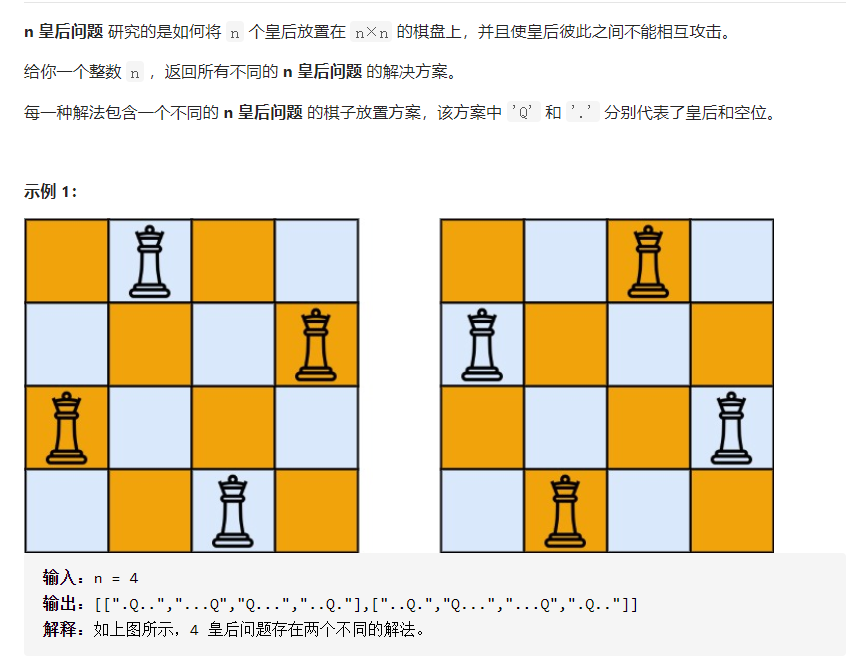N皇后的约数条件：
不能同行不能同列不能同斜线
图解：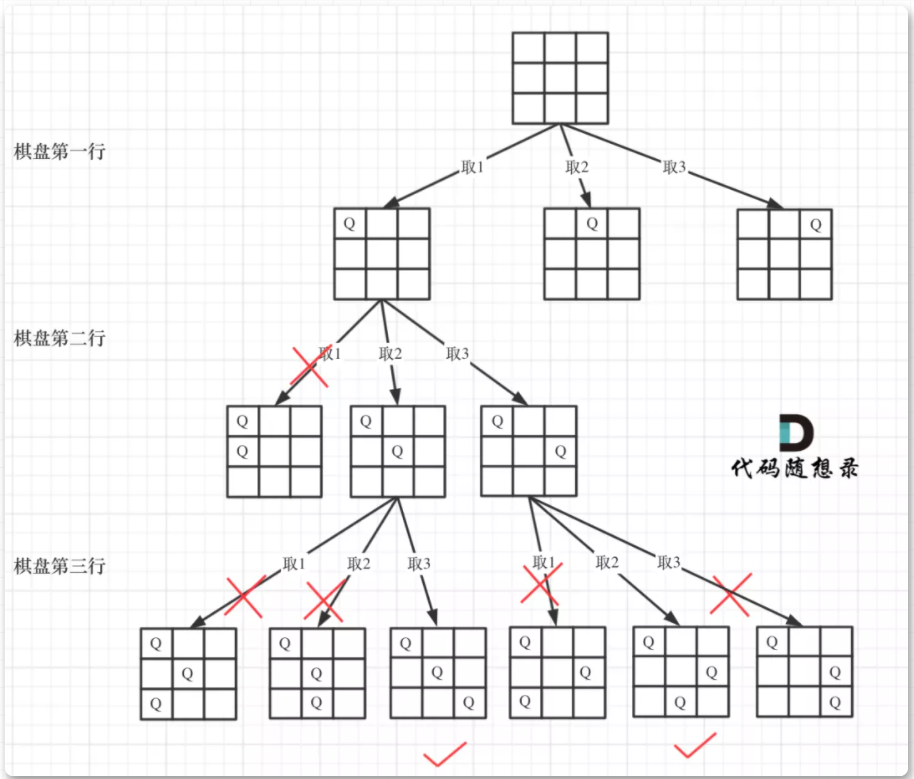这样一看，N皇后问题就清晰多了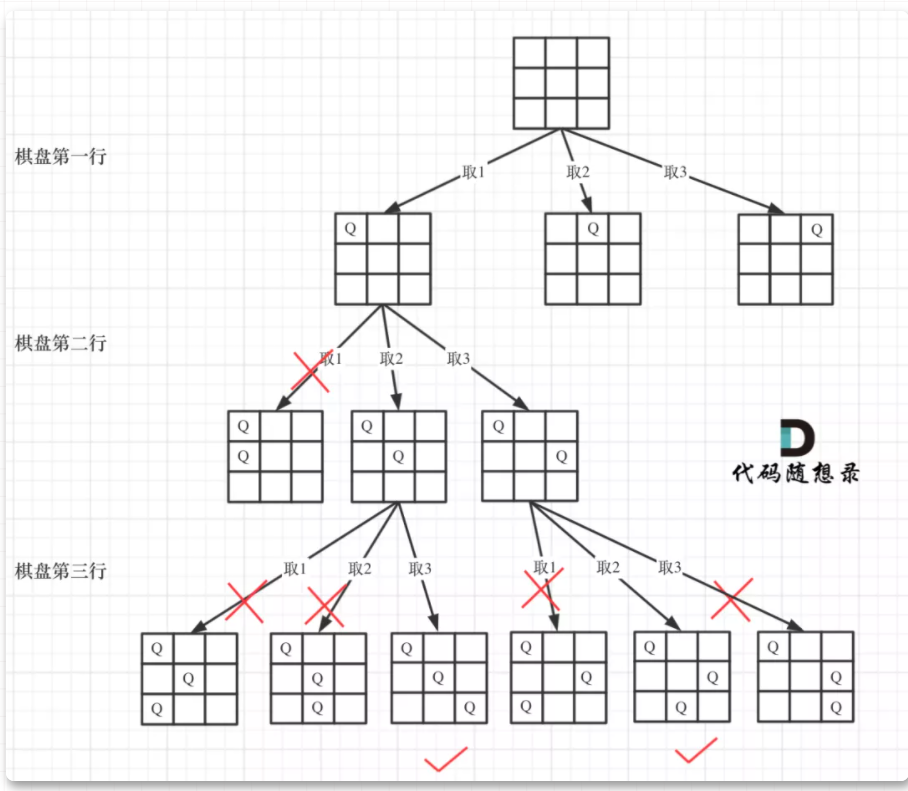void backtracking(参数)
{
if (row == n)
{
存放结果;
return;
}
for (节点展开)
{
if(isValid(row, col, chessboard, n))
{
//设置皇后
chessboard[row][col] = 'Q';
//递归下一层
backtrack(n, row + 1, chessboard);
//回溯,撤销皇后
chessboard[row][col] = '.';
}
}
}

完整代码：
class Solution {
public:
vector<vector<string>> res;
vector<vector<string>> solveNQueens(int n) {
//初始化n x n的棋盘
vector<string> chessboard(n, string(n, '.'));
//row是行
int row = 0;
backtrack(n, 0, chessboard);
return res;
}
bool isValid(int row, int col, vector<string>& chessboard, int n)
{
//检验同一列是否有皇后
for(int i = 0; i < row; i++)
{
if('Q' == chessboard[i][col])
{
return false;
}
}
//检验左对角线是否有皇后
for(int i = row - 1, j = col - 1; i >= 0 && j >= 0; i--,j--)
{
if(chessboard[i][j] == 'Q')
{
return false;
}
}
//检测右对角线是否有皇后
for(int i = row - 1, j = col + 1; i >= 0 && j <= n - 1; i--, j++)
{
if(chessboard[i][j] == 'Q')
{
return false;
}
}
//合理
return true;
}
void backtrack(int n, int row, vector<string>& chessboard)
{
//走到最后一行
if(row == n)
{
res.push_back(chessboard);
return;
}
for(int col = 0; col < n; col++)
{
//检测该位置是否合理
if(isValid(row, col, chessboard, n))
{
//设置皇后
chessboard[row][col] = 'Q';
//递归下一层
backtrack(n, row + 1, chessboard);
//回溯,撤销皇后
chessboard[row][col] = '.';
}
}
}
};

转载：https://mp.weixin.qq.com/s?__biz=MzUxNjY5NTYxNA==&mid=2247485624&idx=1&sn=d560c3a277e1badedc0fa05b8effae87&scene=21#wechat_redirect【代码随想录】
展开全文leetcode
• 此过程使用回溯算法求出在一个n*n棋盘上放置n皇后，使其任意两个皇后即不同行，也不同列，也不在同一斜角线上
• 文章目录N皇后问题描述回溯算法简介回溯算法对问题的要求回溯算法的思想回溯算法的模板使用回溯算法求解N皇后问题 N皇后问题描述 在一个N * N的国际象棋棋盘上需要放置N个皇后，放置时要保证这些皇后不能攻击彼此，...


文章目录
N皇后问题描述回溯算法简介回溯算法对问题的要求回溯算法的思想回溯算法的模板
使用回溯算法求解N皇后问题

N皇后问题描述
在一个N * N的国际象棋棋盘上需要放置N个皇后，放置时要保证这些皇后不能攻击彼此，也就是要保证在同一行、同一列、同一斜线上都只有一个皇后。该问题要求求出所有满足要求的放置方案。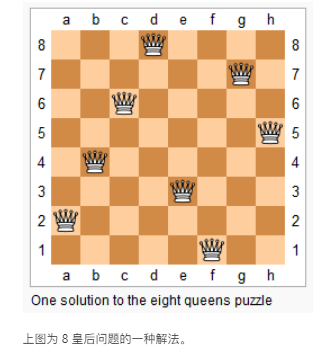回溯算法简介
回溯算法对问题的要求
当一个问题满足：
多步：需要多步来完成多选：每一步有多种决策选择，并且你无法预见哪个选择会使你得到解，哪个选择会使你得不到解瞻前：后续步骤会依赖于先前步骤的选择
这个问题就可以用回溯算法来解决。
拿求解迷宫路径的问题进行比对：
要求1：寻找迷宫路径时，每一步操作就确定路径上的一个点，需要多步来确定一条完整的路径要求2：当你在当前步骤确定路径的下一个节点时，你可能有很多节点可选，并且你不知道选哪个节点才是可以求得最终解的要求3：你在当前步骤的候选节点是由你之前步骤确定的路径决定的
所以求解迷宫问题可以通过回溯算法来解决。读者可以怎么样可以让N皇后问题也符合回溯算法的要求，没有思路可以看下文使用回溯算法求解N皇后问题”的部分。
回溯算法的思想
回溯算法用到的思想是：从一条路往前走，能进则进，不能进则退回来，换一条路再试，以此来把所有的可能性都考虑一遍。总结起来也就是深度优先搜索。
回溯算法的模板
回溯算法是由递归函数实现的，这个递归函数的基本骨架如下：
void backtrack(resultStack, arg1, arg2, ...){
// 当满足题目的某种要求时，说明此时找到了问题的一个解
if (some condition){
// 在这里根据题目要求做出相关操作
// 这里的操作一般是将resultStack复制一份，加入到所有解的集合中
some operations;

// 直接返回
return;
}

// candidates 表示"要求2"中提到的多种候选决策
// 在这里通过for循环遍历所有的候选决策
for (candidate : candidates){
// 当候选条件不符合要求时，跳过这个条件
if (candidate does not satisfy some requirements){
continue;
}

// 下面三行是这个函数的核心，体现了回溯算法能进则进的思想
// 先把当前候选条件加入结果栈中，然后进入下一步，
// 从下一步中返回后，将当前候选条件出栈，把结果栈恢复到之前的状态，
// 以便考虑下一个candidate（for循环的下一个元素）
resultStack.push(candidate);
backtrack(resultStack, arg1, arg2);
resultStack.pop();
}
}

使用回溯算法求解N皇后问题

题目要求请看这里： https://leetcode-cn.com/problems/n-queens/
如何确定思路呢？可以从回溯算法的要求出发：多步、多选、瞻前。首先要把问题分解成一个多步可解的情形，我们可以这样想：棋盘的每行只能而且必须放一个皇后，只要把所有行都放上一个皇后，放置方案就出来了，所以此时回溯算法的每一步就是：在当前行中放置一个皇后。然后我们就可以看这样满不满足回溯算法的要求2和要求3了，每一行有N列，每一列都是一个决策选择，所以满足要求2；在选择当前行要在哪一列放置皇后时，要保证该位置不会和之前放置的皇后在同一列、或者同一斜线上，所以满足要求3。
这样一来解决方案其实很简单了，只要套上面的模板就可以了：
import java.util.*;

class Solution {
// 用二维数组表示棋盘
private char[][] board;
// N皇后问题的N
private int n;
// 所有可行的放置方案的集合
private List<List<String>> solutions = new ArrayList<>();

// 主函数
public List<List<String>> solveNQueens(int n) {
this.n = n;
// 初始化棋盘，并且把每一个单元格都设置为'.'，表示棋盘是空的，没有皇后
this.board = new char[n][n];
for (int i = 0; i < n; i++){
for (int j = 0; j < n; j++){
board[i][j] = '.';
}
}

// 进入回溯函数
backtrack(new ArrayList<>(), new ArrayList<>(), new ArrayList<>(), 0);
return solutions;
}

// 实现回溯算法的函数
// usedCols 表示使用过的列号，列号从0开始
// usedDianonals1 表示使用过的、方向为从左上到右下的 斜线号
// usedDianonals2 表示使用过的、方向为从右上到左下的 斜线号
// row 表示当前步骤的行号，行号从0开始
private void backtrack(List<Integer> usedCols, List<Integer> usedDiagonals1, List<Integer> usedDiagonals2, int row){
// 行号从0开始，所以棋盘的最后一行的标号是n-1，
// 当行号为n时，说明所有的行都已经放置了正确位置的皇后，可以添加解了。
if (row == n){
return;
}

// 遍历当前行的所有列
for (int j = 0; j < n; j++){
if (usedCols.contains(j) || usedDiagonals1.contains(row - j) || usedDiagonals2.contains(row + j)){
continue;
}

board[row][j] = 'Q';
backtrack(usedCols, usedDiagonals1, usedDiagonals2, row + 1);
board[row][j] = '.';
usedCols.remove(usedCols.size() - 1);
usedDiagonals1.remove(usedDiagonals1.size() - 1);
usedDiagonals2.remove(usedDiagonals2.size() - 1);
}

}

List<String> solution = new ArrayList<>();
for (int i = 0; i < n; i++){
StringBuilder builder = new StringBuilder();
for (int j = 0; j < n; j++){
builder.append(board[i][j]);
}
}

}
}

展开全文• NQueens_Backtracking 回溯算法解决N皇后问题Java
• 回溯算法实现N皇后问题,由用户输入皇后的个数,输出全部的解,和解的总个数 环境VC6.0
• ## 回溯算法之N皇后问题

千次阅读 多人点赞 2021-04-24 20:55:54
皇后问题（英文：Eight queens），是由国际西洋棋棋手马克斯·贝瑟尔于1848年提出的问题，是回溯算法的典型案例。 问题表述为：在8×8格的国际象棋上摆放8个皇后，使其不能互相攻击，即任意两个皇后都不能处于同一...
问题描述
什么是皇后问题(有一定了解可以直接跳过这个部分看求解部分哦)

八皇后问题（英文：Eight queens），是由国际西洋棋棋手马克斯·贝瑟尔于1848年提出的问题，是回溯算法的典型案例。 问题表述为：在8×8格的国际象棋上摆放8个皇后，使其不能互相攻击，即任意两个皇后都不能处于同一行、同一列或同一斜线上，问有多少种摆法。高斯认为有76种方案。1854年在柏林的象棋杂志上不同的作者发表了40种不同的解，后来有人用图论的方法解出92种结果。如果经过±90度、±180度旋转，和对角线对称变换的摆法看成一类，共有42类。计算机发明后，有多种计算机语言可以编程解决此问题。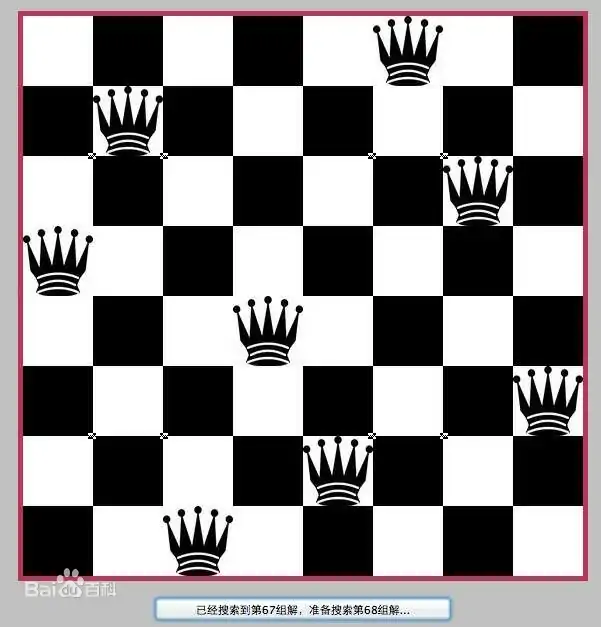一起看看经典教材 计算机算法设计与分析 对该问题的描述：
在 n × n 棋盘上放彼此不受攻击的n个皇后。按照国际象棋规则，皇后可以攻击 同行、同列、同一斜线 的棋子。等价于在 n × n 格的棋盘上放置 n 个皇后，任何 2 个皇后不放在 同一行 或 同一列 或 同一斜线 上。
解题思路
由于皇后的位置受到上述三条规则约束，我们必须通过一些技术手段来判断当前皇后的位置是否合法。
1.皇后的编号从 0 ~ N - 1 （N表示皇后的数量），这样编号的想法很简单：数组下标从0开始（这样方便后续对其位置的说明）。
2.使用一维数组 putInf 对每一行皇后的存放位置进行保存，因此得到解向量 (putInf, putInf, putInf, … , putInf[N - 1])，putInf[i] 表示第 i 个皇后被放置到了第 putInf[i] + 1 列上（putInf数组中存储的是列号，范围为 0 ~ N - 1）;
3.第二个条件：各皇后不同列， N 皇后放在 N x N 的棋盘上，那么每一列最多且必须放置一个皇后，这里我用了一个 used数组 对每一列的摆放情况进行记录， used[i] = true 表示 第 i 列 已经放置了皇后，used[i] = false 表示第i列暂未放置皇后，这样我们可以保证不在一列上放置多个皇后，也就能满足 各皇后不同列 的规则。
4.各皇后不能处于同一对角线位置：假设两皇后位置坐标分别为(i, j) 、（l, k），那么根据直线斜率公式：
(i - l) / (j - k) = 1 求解得 i - l == j - k ①(i - l) / (j - k) = -1 求解得 i - l == k - j ② 这两种情况出现时表明处于同一对角线，那么要满足摆放规则就必须满足 | i - l | != | j - k | （“| |” 表示绝对值）
解空间树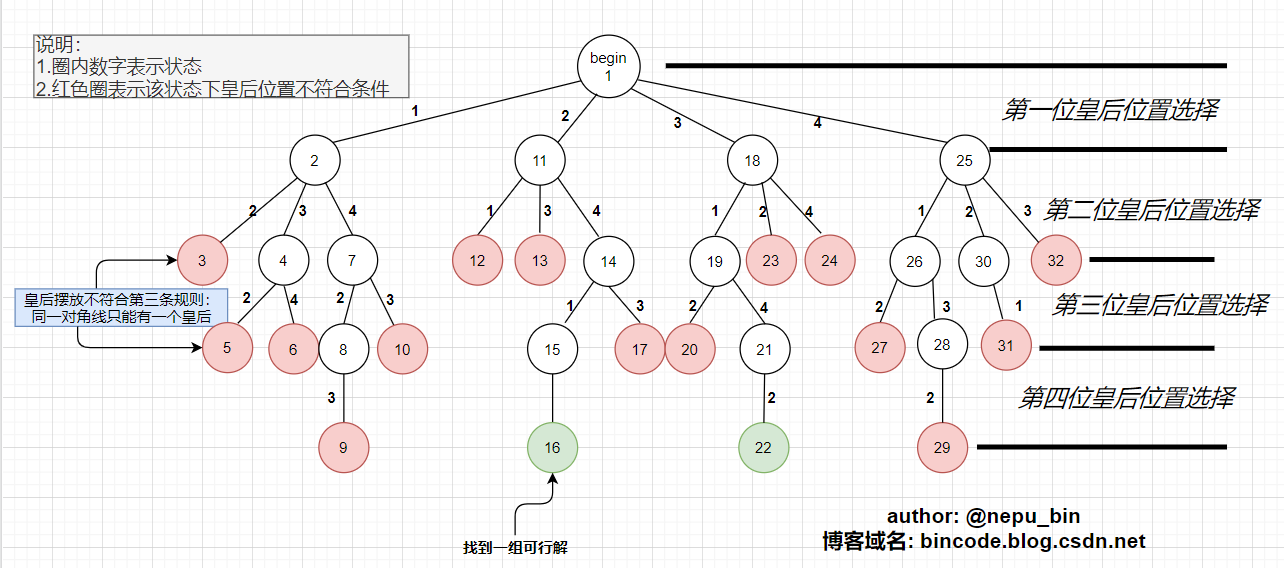实现代码
#include <iostream>
#include <vector>
using namespace std;

#define N 4 //N皇后
vector<int> putInf;//每一行皇后的置放位置情况
//不同行 不同列 不同斜线 |ri - rj| != |ci - cj| 第1行与
vector<int> used(N, 0);//每一列只能有一个皇后，记录每一列的状态
vector<vector<int>> ans;//存储可行方案
int curRow = 0;//当前待放皇后的行数

/*            正置放皇后行↓ 置放列↓              */
bool judgeLegalPut(int& curRow, int col) {//判断在curRow行的col列放置皇后是否合法
for (int i = curRow - 1; i >= 0; i--) {
//我们的解空间树已经去除一行一列置放相同元素
//（每一个皇后被放在不同行以及不同列）的情况
//因此我们只需要判断皇后是否成斜线即可
if (curRow - i == abs(col - putInf[i])) {
//当前位置与之前的皇后处于同一斜线上
return false;
}
}
return true;
}

void queensAssign(int curRow) {
if (curRow >= N) {//递归到叶子节点，递归结束，收集结果
ans.push_back(putInf);
return;
}

//i : 当前行皇后准备放的列数
for (int i = 0; i < N; ++i) {//curRow行i列的位置
if (used[i]) continue;//位置被使用过，直接跳过
//这样满足了不处于同一列的显条件 类似于全排列
if (judgeLegalPut(curRow, i)) {
//当前位置置放与之前不冲突 将皇后加入
used[i] = true;
putInf.push_back(i);
queensAssign(curRow + 1);
used[i] = false;//撤销之前的状态
putInf.pop_back();
}
}
}

void printChessBoard(vector<int>& vec) {//输出模拟棋盘
cout << endl;
for (int i = 0; i < N; i++) {
for (int j = 0; j < N; j++) {
if (j != vec[i])
cout << "○";
else
cout << "●";
}cout << endl;
}cout << endl;
}
/// <author>
/// nepu_bin
/// <博客域名>
/// bincode.blog.csdn.net
int main() {
queensAssign(0);
int n = 1;
cout << N << "皇后问题,方案如下:\n" << endl;
for (vector<vector<int>>::iterator it = ans.begin(); it != ans.end(); it++) {
cout << "第" << n++ << "种放置方案, 皇后被放于 " << endl;
for (int i = 0; i < it->size(); i++) {
cout << (*it)[i] + 1 << "  ";
}cout <<"列" << endl;
cout << endl << "棋盘布局如下↓" << endl;
printChessBoard(*it);
}
return 0;
}

运行效果
四皇后问题运行截图：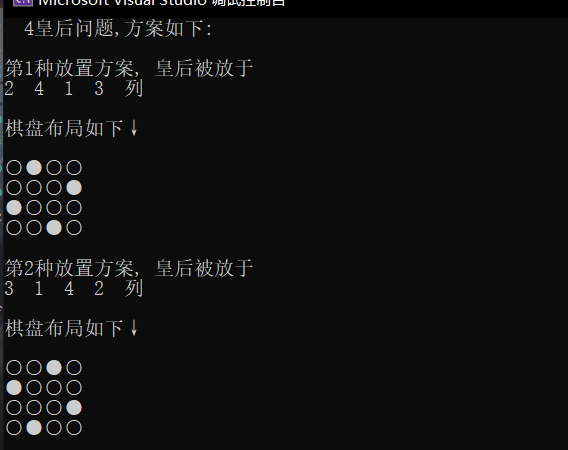通过修改宏定义 N 可以得到不同数量皇后问题的解答~~~ 八皇后求解（部分解）：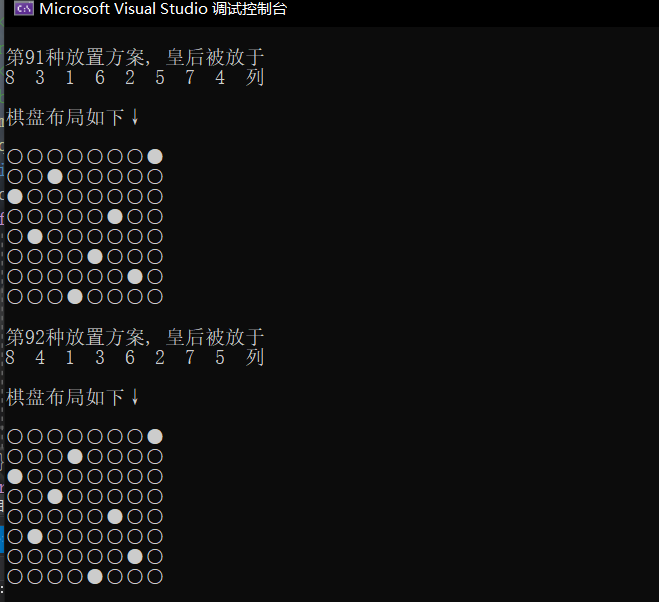子集树与排列树
附上子集树 and 排列树的定义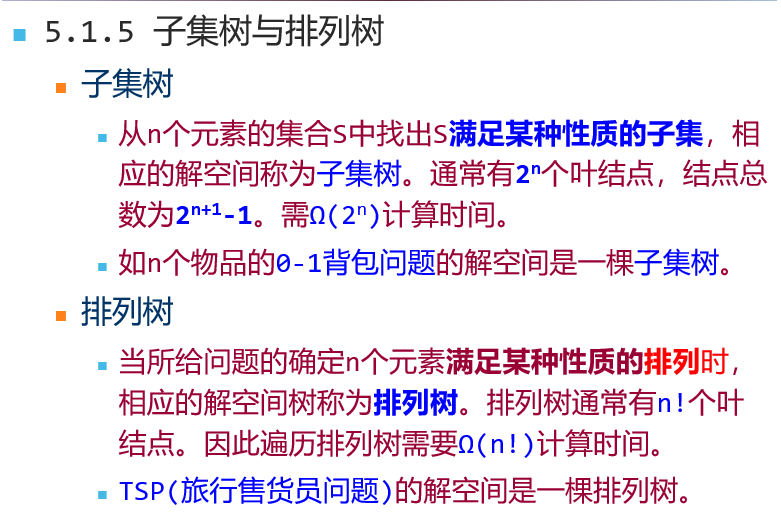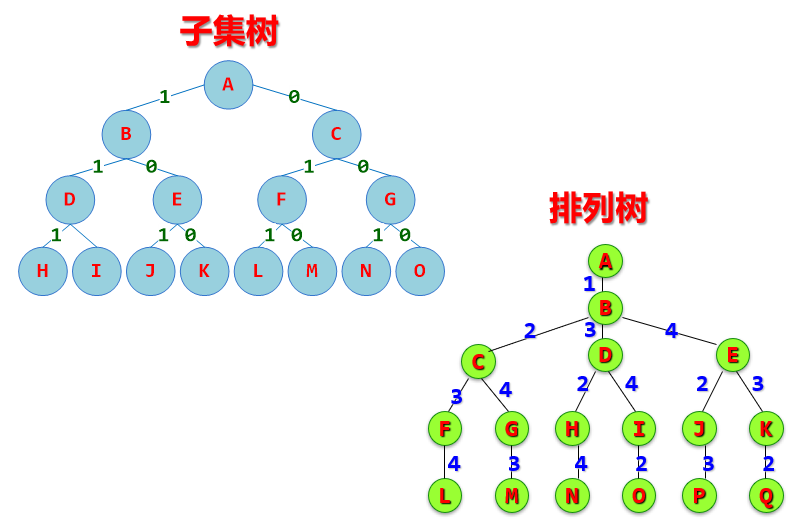在了解过该问题之后便可以开始着手力扣上的N皇后问题，在这里贴一下实现代码：
LeetCode必刷经典: n 皇后问题
n 皇后问题，研究的是如何将 n 个皇后放置在 n×n 的棋盘上，并且使皇后彼此之间不能相互攻击。 给你一个整数 n ，返回所有不同的 n 皇后问题 的解决方案。 每一种解法包含一个不同的 n 皇后问题 的棋子放置方案，该方案中 ‘Q’ 和 ‘.’ 分别代表了皇后和空位。
链接：https://leetcode-cn.com/problems/n-queens 思路与上面完全一致，直接上实现代码：
class Solution {
public:
vector<vector<string>> res;
vector<int> put;//记录每个皇后放置的位置:i行put[i]列
vector<string> solution;
vector<bool> haveQ;//记录每一列位置上皇后的摆放情况
//推导解空间树: 排列树、解集树
//N皇后问题为排列树结构，每个皇后都需要放置 depth变量记录当前正摆放皇后的位置
void dfs(int depth, int& n) {
if (depth >= n) {
res.push_back(solution);
return;//此时已经完成所有皇后的摆放
}
for (int i = 0; i < n; i++) {//i表示列值
if (haveQ[i]) continue;//当前列已经有皇后

haveQ[i] = true;
solution[depth][i] = 'Q';
put[depth] = i;

int j;
//当前列无皇后，试探性摆放
for (j = 0; j < depth; j++) {
//检测前 depth - 1行是否发生冲突
if (abs(j - depth) == abs(put[j] - i))
break;
}
if (j >= depth) {
//检测通过，继续深入
dfs(depth + 1, n);
}
//检测失败，撤销之前的操作
haveQ[i] = false;
solution[depth][i] = '.';
}
}

vector<vector<string>> solveNQueens(int n) {
if (n == 1) return { {"Q"} };
string str;
str.assign(n, '.');//初始化n个'.'的字符串

//保证横行纵行、斜线都不存在皇后
//abs(y - cury) = abs(i - curi)
haveQ.resize(n, false);
solution.resize(n, str);
put.resize(n, -1);//初始化3个容器

dfs(0, n);
return res;
}
};

在这里的巧妙之处是：
利用了循环的顺序性消除了第一层限制: 同一行中不可以存在两个皇后，由于是顺序遍历，依次摆放皇后且每次只放置一个，因此该条件我们很容易实现。第二个条件是同一列上不可以有两个及以上的皇后，在代码中使用了put数组，记录了每个皇后的摆放位置，利用了哈希映射的原理(put数组的下标( 0~put.size - 1) 对应着每个皇后，下标对应存储的值则表示了此位皇后摆放在了哪一列，打个比方: 下标i表示了第i位皇后(假设皇后的编号从零开始)， put[i]则表示第i位皇后被放在了put[i] 列；这么做的好处是为了实现有哈希表一样的查询效率O(1)。第三条限制则是在回溯算法的核心部分体现：
//当前列无皇后，试探性摆放
for (j = 0; j < depth; j++) {
//检测前 depth - 1行是否发生冲突
if (abs(j - depth) == abs(put[j] - i))
break;
}
if (j >= depth) {
//检测通过，继续深入
dfs(depth + 1, n);
}
//检测失败，撤销之前的操作
haveQ[i] = false;
solution[depth][i] = '.';

在模拟放置皇后之后进行了检查，通过与之前摆放的皇后位置比较是否出现在一条斜线上，若存在，则不在继续往下深入递归。展开全文c++ c语言
• 这是一个N皇后问题回溯算法改进版，c语言版，对于学习算法设计的同学可能会用到
• 回溯算法基本思想： 回溯法（探索与回溯法）是一种选优搜索法，又称为试探...八皇后问题： 是回溯算法的典型案例。该问题是国际西洋棋棋手马克斯·贝瑟尔于1848年提出：在8×8格的国际象棋上摆放八个皇后，使其不能互
• 源代码 #include <cstdio> #include <cmath> #include <algorithm>...using namespace std;... //皇后的位置用列值来表示，下标为行数，值为列 bool isPlace(int k) { for(int...C/C++
• n皇后问题等价于在n×n格的棋盘上放置n个皇后，任何两个皇后不放在同一行或同一列或同一斜线上。 编程要求：找出一个n×n格的棋盘上放置n个皇后并使其不能互相攻击的所有方案。 输入输出样例 ...
• 分别用随机算法回溯法求解N皇后问题 附有详细C++源代码
• 要在n×n的国际象棋棋盘中放入n皇后，使任意两个皇后都不能互相吃掉。
•C语言
• 回溯算法实际上一个类似枚举的搜索尝试过程，主要是在搜索尝试过程中寻找问题的解，当发现已不满足求解条件时，就“回溯”返回，尝试别的路径。许多复杂的，规模较大的问题都可以使用回溯法，有“通用解题方法”的...
• n问题要求在一个n×n格的棋盘上放置n皇后，使得它们彼此不受攻击。按照国际象棋的规则，一个皇后可以攻击与之处在同一行或同一列或同一条斜线上的其他任何棋子。因此，n问题等价于要求在一个n×n格的棋盘上...
• 学习回溯算法问题，最为经典的问题我想应该就是八皇后问题了。 一、适用范围 　回溯算法应用的范围当然是很多了，那么归纳一下：如果一个问题中，没有很好的数学模型来解决，或者有数学模型解决，但是很难实现，...
• AI_Project:使用差分进化和回溯算法解决n皇后问题
•  寻找问题的解的一种可靠的方法是首先列出所有候选解，然后依次检查每一个，在检查完所有或部分候选解后，即可找到所需要的解。理论上，当候选解数量有限并且通过检查所有或部分候选解能够得到所需解时，上述方法是......